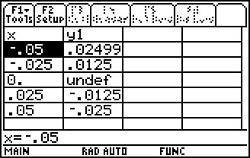Activities

••• Subject Area

• Math: Calculus: Derivatives

• Author9-12

45 Minutes

• Device
• TI-89 / TI-89 Titanium

The Trigonometric DerivativeActivity Overview

Students will derive the derivative of the functions y = sin(x), y = cos(x), and y = tan(x), and work with the derivatives of both y = sin(u), and y = cos(u).

Before the Activity

Download the attached PDF and look over the information on the first page. Distribute the student worksheet for use during the activity.

During the Activity

Discuss the material from the activity pages with students as needed.

After the Activity

Encourage students to summarize what they have learned from completing the activity. If desired, discuss the extension with students.